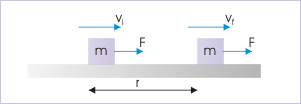## Kinetic Energy and Work-Energy Theorem

The work-energy theorem states that the work done by all forces acting on a particle equals the change in the particle’s kinetic energy.

### Learning Objectives

Outline the derivation of the work-energy theorem

### Key Takeaways

#### Key Points

• The work W done by the net force on a particle equals the change in the particle’s kinetic energy KE: $\text{W}=\Delta \text{KE}=\frac{1}{2} \text{mv}_\text{f}^2-\frac{1}{2} \text{mv}_\text{i}^2$.
• The work-energy theorem can be derived from Newton’s second law.
• Work transfers energy from one place to another or one form to another. In more general systems than the particle system mentioned here, work can change the potential energy of a mechanical device, the heat energy in a thermal system, or the electrical energy in an electrical device.

#### Key Terms

• torque: A rotational or twisting effect of a force; (SI unit newton-meter or Nm; imperial unit foot-pound or ft-lb)

### The Work-Energy Theorem

The principle of work and kinetic energy (also known as the work-energy theorem) states that the work done by the sum of all forces acting on a particle equals the change in the kinetic energy of the particle. This definition can be extended to rigid bodies by defining the work of the torque and rotational kinetic energy.Kinetic Energy: A force does work on the block. The kinetic energy of the block increases as a result by the amount of work. This relationship is generalized in the work-energy theorem.

The work W done by the net force on a particle equals the change in the particle’s kinetic energy KE:

$\text{W}=\Delta \text{KE}=\frac{1}{2} \text{mv}_\text{f}^2-\frac{1}{2} \text{mv}_\text{i}^2$

where vi and vf are the speeds of the particle before and after the application of force, and m is the particle’s mass.

### Derivation

For the sake of simplicity, we will consider the case in which the resultant force F is constant in both magnitude and direction and is parallel to the velocity of the particle. The particle is moving with constant acceleration a along a straight line. The relationship between the net force and the acceleration is given by the equation F = ma (Newton’s second law), and the particle’s displacement d, can be determined from the equation:

$\text{v}_\text{f}^2 = \text{v}_\text{i}^2 + 2\text{ad}$

obtaining,

$\text{d}=\frac{\text{v}_\text{f}^2-\text{v}_\text{i}^2}{2\text{a}}$

The work of the net force is calculated as the product of its magnitude (F=ma) and the particle’s displacement. Substituting the above equations yields:

$\text{W}=\text{Fd}=\text{ma}\frac{\text{v}_\text{f}^2-\text{v}_\text{i}^2}{2\text{a}}=\frac{1}{2} \text{mv}_\text{f}^2-\frac{1}{2} \text{mv}_\text{i}^2=\text{KE}_\text{f}-\text{KE}_\text{i}=\Delta \text{KE}$